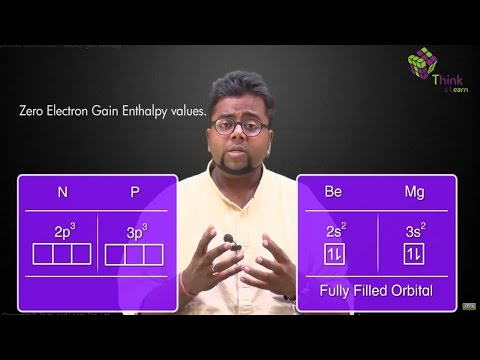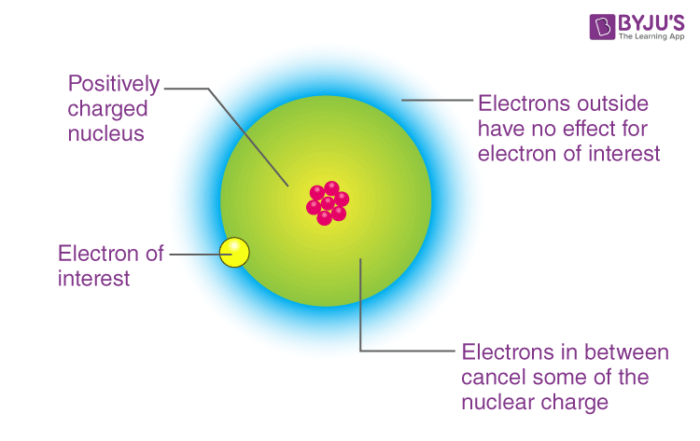# Electron Gain EnthalpyElectron gain enthalpy is sometimes also referred to as Electron affinity although there is a minute difference between them. Electron gain enthalpy is defined as the amount of energy released when an electron is added to an isolated gaseous atom. During the addition of an electron, energy can either be released or absorbed.

Let us consider two metals Magnesium and Sodium. Metals lose electrons to obtain the inert gas configuration. Hence, Sodium and Magnesium atoms will not add electrons easily. Some external energy is needed to add the electron in their atoms. Hence, electron gain enthalpy for metals will be positive. Magnesium atom is small, so the attractive nuclear force will be more on the electrons whereas the size of Sodium atom is comparatively larger than Magnesium atom. Hence, the nuclear force applied on the electrons in the case of Sodium will be less.So, we will apply energy in both the cases but the amount of energy needed in the case of Magnesium will be lesser than that of Sodium because of the help we are receiving from the nuclear charge of Magnesium in attracting the electron. So the electron gain enthalpy in both the cases will be positive, but the atom with a smaller size will have lesser positive electron gain enthalpy. So we can conclude that electron gain enthalpy depends on atomic radius and the nuclear charge.

Now, take two non-metals Sulphur and Chlorine. The electronic configuration of Chlorine is 3p5 and Sulphur has the configuration of 3p4. If we add one electron to these non-metals than Chlorine will accept it first. After acquiring one electron it will achieve its noble gas configuration. After acquiring one electron, the stability of Chlorine will be more.

So it will remove the maximum amount of energy. Hence, the electron gain enthalpy of chlorine is more negative as compared to Sulphur. Let’s verify this by the property of atomic radius size and nuclear charge. The atomic radius of chlorine is smaller than that of Sulphur so the effective nuclear charge on an electron in the case of chlorine will be more than that of Sulphur. Thus, the chlorine will have more negative electron gain enthalpy.

From the above discussion, we can understand that electron gain enthalpy depends on three factors

• Electronic configuration
• Nuclear charge.

Electron affinity means love for electron. It is the negative of the electron gain enthalpy. By using the concepts of thermodynamics, we find a relationship between electron gain enthalpy and electron affinity i.e.

Electron gain enthalpy = electron affinity –

$$\begin{array}{l} \frac 52 \end{array}$$
RT

where, R= universal gas constant

T= temperature in Kelvin scale

Exception in Electron Gain Enthalpy:

• In the case of Chlorine and Fluorine, Chlorine has a higher negative electron gain enthalpy value.
• In between Sulphur and Oxygen, Sulphur has a higher negative value than oxygen.
Test your knowledge on Electron Gain Enthalpy

1. Thanks for clarifying the dout

2. Thanks

3. Thx

4. shaharban

5. Thank you so much !!!!!

6. Michelle

Thank you so much🤓

7. Like

8. Thanks so much

9. ravindi

thankyou very much Clearly explained